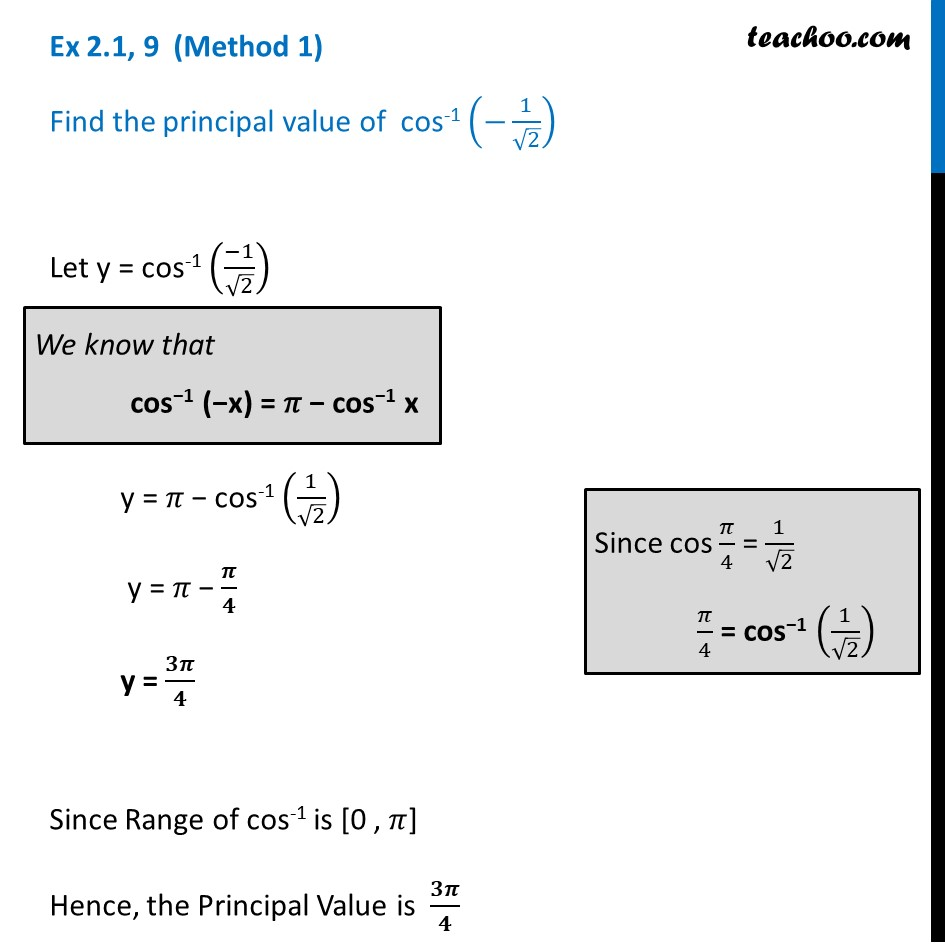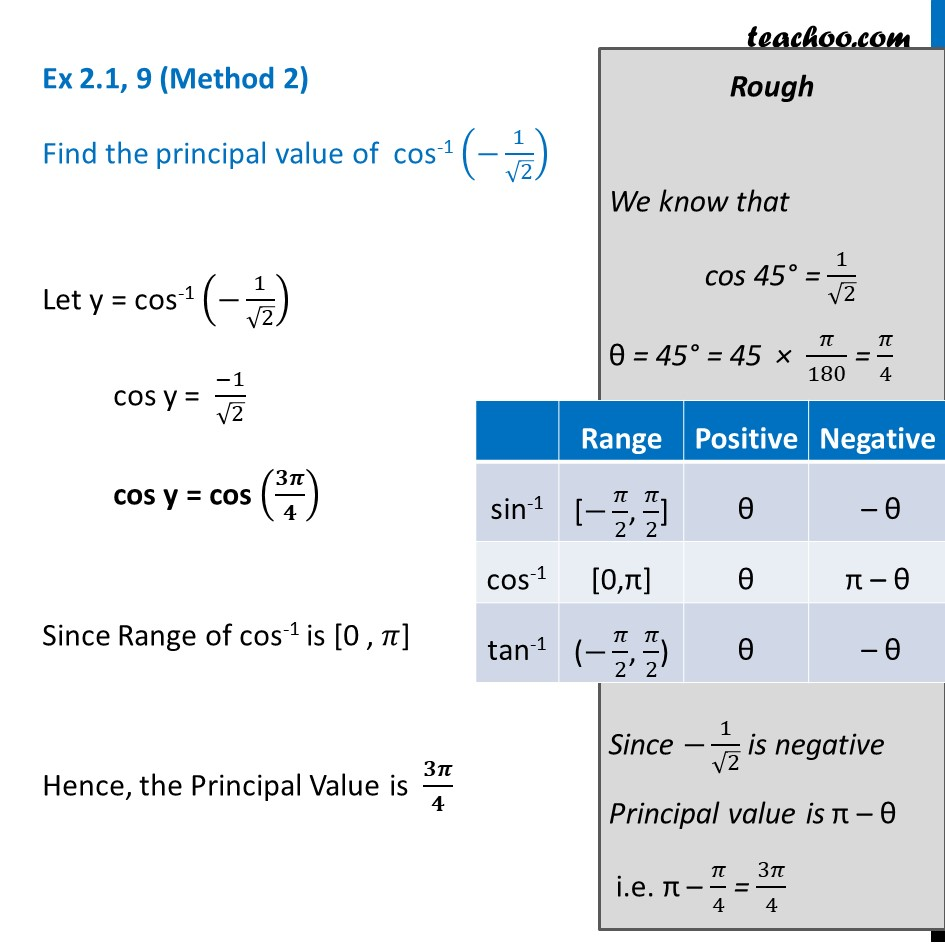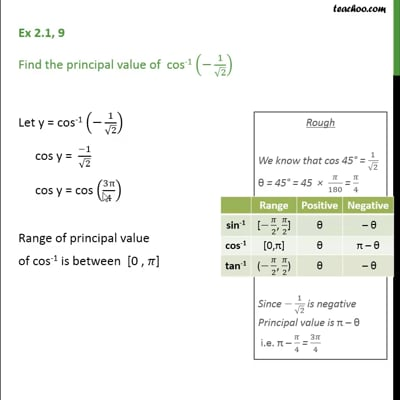Ex 2.1

Chapter 2 Class 12 Inverse Trigonometric Functions
Serial order wiseThis video is only available for Teachoo black users

Solve all your doubts with Teachoo Black (new monthly pack available now!)

### Transcript

Ex 2.1, 9 (Method 1) Find the principal value of cos-1 (−1/√2) Let y = cos-1 ((−1)/√2) y = 𝜋 − cos-1 (1/√2) y = 𝜋 − 𝝅/𝟒 y = 𝟑𝝅/𝟒 Since Range of cos-1 is [0 , 𝜋] Hence, the Principal Value is 𝟑𝝅/𝟒 We know that cos−1 (−x) = 𝜋 − cos−1 x Since cos 𝜋/4 = 1/√2 𝜋/4 = cos−1 (1/√2) Ex 2.1, 9 (Method 2) Find the principal value of cos-1 (−1/√2) Let y = cos-1 (−1/√2) cos y = (−1)/√2 cos y = cos (𝟑𝝅/𝟒) Since Range of cos-1 is [0 , 𝜋] Hence, the Principal Value is 𝟑𝝅/𝟒 Rough We know that cos 45° = 1/√2 θ = 45° = 45 × 𝜋/180 = 𝜋/4 Since −1/√2 is negative Principal value is π – θ i.e. π – 𝜋/4 = 3𝜋/4